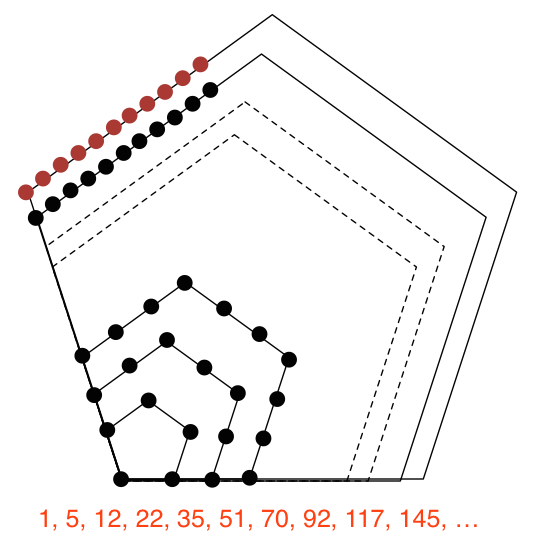# Pentagonal Numbers - Finding The Formula

Probability Level 1Which of the following functions satisfy $f(n+1) - f(n) = 3n+1$ and $f(1) = 1$?

Do you understand why this gives us the formula for the $n$th Pentagonal Number?

This question is part of Calvin's set What Makes A Number Pentagonal?

×